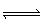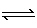Name:    Practice Test 2A

Multiple Choice

1.

Which of the following 0.1-M solutions is most acidic?
 A) pH = 7.22 B) pH = 8.34 C) pH = 11.88 D) None of the above; these solutions are all basic

2.

What is the [H+] for a solution that has pOH = 5.640?
 A) 4.27 x 10–11 M B) 2.34 x 10–4 M C) 4.37 x 10–9 M D) 2.29 x 10–6 M

3.

Benzoic acid (C6H5CO2H) is 1.0% ionized in a 0.010 M solution.  What is the value of Ka for this acid?
 A) 1.0x10–3 B) 1.0x10–6 C) 1.0x10–8 D) 1.0x10–4

4.

Which of the following pairs has the stronger acid listed first?
 A) H2AsO3 and H2AsO4 B) HI and HBr C) HClO and HClO2 D) H2S and HCl

5.

The following equilibrium is established with the concentration of the products greater than the reactants:

H2C2O4 (aq)  +   H2PO4HC2O4  (aq)  +  H3PO4 (aq)

Which of the following statements is true?
 A) The HC2O4– anion is a stronger base than H2PO4– B) H2C2O4 is a weaker acid than H3PO4 C) H3PO4 ia a weaker acid than H2C2O4 D) H2PO4– anion is a stronger acid than H2C2O4

6.

What is the pH of a 0.0085 M KOH solution?
 A) 11.93 B) 4.77 C) 9.23 D) 2.07

7.

Which of the following is NOT a conjugate acid-base pair?
 A) H3O+ / H2O B) HNO2 / NO2– C) H2S / S2– D) HSO4– / SO42–

8.

As the pH of a solution increases, its acidity ______________; as the pOH of a solution increases, its acidity_______________
 A) increases; increases B) increases; decreases C) decreases; increases D) decreases; decreases

9.

A 0.20 M solution of a weak monoprotic acid has a pH of 3.00.  The ionization constant of this acid is
 A) 5.0x10–3 B) 2.0x10–7 C) 5.0x10–7 D) 5.0x10–6

10.

Each of the following can act as both a Bronsted-Lowry acid and base except
 A) HCO3– B) NH4+ C) HS– D) H2PO4–

11.

Which of the following correctly lists a conjugate acid-base pair, respectively,  in the reaction shown below:

HSO4  +  HCO3SO42– +  H2CO3
 A) HCO3– and SO42– B) HSO4– and HCO3– C) HSO4– and SO42– D) HCO3– and H2CO3

12.

What is the pH of a 0.050 M trimethylamine, (C2H5)3N, solution? (Kb = 5.3x10-4)
 A) 8.68 B) 11.7 C) 10.5 D) 2.3

13.

The equilibrium constant for the reaction shown below is greater than 1.0.  Which of the following statements about the strength of the acids and bases is correct?

H2PO4  +  HBO32–HPO4 +  H2BO3
 A) H2PO4– is weaker acid than H2BO3– and HPO4– is weaker base than HBO32– B) H2PO4– is stronger acid than H2BO3– and HPO4– is stronger base than HBO32– C) H2PO4– is stronger acid than H2BO3– and HPO4– is weaker base than HBO32– D) H2PO4– is weaker acid than H2BO3– and HPO4– is stronger base than HBO32–

14.

The term “Ka for the ammonium ion, NH4+”, refers to which equation?
 A) NH4+ (aq) + OH– (aq)NH3 (aq) + H2O (l) B) NH3 (aq) + H2O (l)NH4+ (aq) + OH– (aq) C) NH3 (aq) + H3O+ (aq)NH4+ (aq) + H2O (l) D) NH4+ (aq) + H2O (l)NH3 (aq) + H3O+ (aq)

15.

Which of the following species in in the greatest concentration in a 0.10-M solution of H2SO4?
 A) HSO4– B) SO42– C) H3O+ D) All species are in equilibrium and therefore have the same concentrations

16.

What is the pH of a 0.0100 M sodium benzoate solution?  Kb (C7H5O2) = 1.5x10–10
 A) 5.91 B) 8.09 C) 0.38 D) 13.62